# Electronics and Communication Engineering - Microwave Communication

### Exercise :: Microwave Communication - Section 6

41.

Assertion (A): Inter-digitated geometry is widely used for microwave transistors.

Reason (R): Tuning at input and output of transistor uses MOS chip capacitors.

 A. Both A and R are correct and R is correct explanation of A B. Both A and R are correct but R is not correct explanation of A C. A is correct but R is wrong D. A is wrong but R is correct

Answer: Option B

Explanation:

No answer description available for this question. Let us discuss.

42.

For a 1 cm x 2 cm air filled rectangular waveguide the maximum frequency should be less than about

 A. 1000 MHz B. 5000 MHz C. 14000 MHz D. 140000 MHz

Answer: Option C

Explanation:

No answer description available for this question. Let us discuss.

43.

Hollow waveguides are used as transmission lines at frequencies

 A. above 1 GHz B. above 5 GHz C. above 10 MHz D. above 1 MHz

Answer: Option B

Explanation:

No answer description available for this question. Let us discuss.

44.

If rv is reflection coefficient and VSWR is voltage standing wave ratio, then

 A.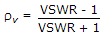B.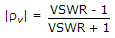C.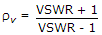D.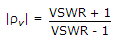Answer: Option B

Explanation:

No answer description available for this question. Let us discuss.

45.

In the given figure the equivalent circuit of a T attenuator. If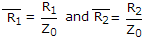the condition for matched attenuator is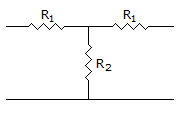A.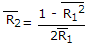B.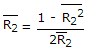C.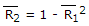D.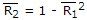Answer: Option A

Explanation:

No answer description available for this question. Let us discuss.

#### Current Affairs 2021

Interview Questions and Answers